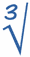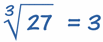# Cubes and Cube Roots

To understand cube roots, first we must understand cubes ...

## How to Cube A Number

To cube a number, just use it in a multiplication 3 times ...

### Example: What is 3 Cubed?

 3 Cubed == 3 × 3 × 3 = 27

Note: we write "3 Cubed" as 33
(the little 3 means the number appears three times in multiplying)

## Cubes From 03 to 63

 0 cubed = 03 = 0 × 0 × 0 = 0 1 cubed = 13 = 1 × 1 × 1 = 1 2 cubed = 23 = 2 × 2 × 2 = 8 3 cubed = 33 = 3 × 3 × 3 = 27 4 cubed = 43 = 4 × 4 × 4 = 64 5 cubed = 53 = 5 × 5 × 5 = 125 6 cubed = 63 = 6 × 6 × 6 = 216

## Cube Root

A cube root goes the other direction:

3 cubed is 27, so the cube root of 27 is 3

 327

The cube root of a number is ...
... a special value that when cubed gives the original number.

The cube root of 27 is ...
... 3, because when 3 is cubed you get 27.Note: When you see "root" think "I know the tree, but what is the root that produced it?" In this case the tree is "27", and the cube root is "3".

Here are some more cubes and cube roots:4 64 5 125 6 216

### Example: What is the Cube root of 125?

Well, we just happen to know that 125 = 5 × 5 × 5 (if you use 5 three times in a multiplication you will get 125) ...

... so the cube root of 125 is 5

## The Cube Root SymbolThis is the special symbol that means "cube root", it is the "radical" symbol (used for square roots) with a little three to mean cube root.

You can use it like this:(we say "the cube root of 27 equals 3")

## You Can Also Cube Negative Numbers

Have a look at this:

When we cube +5 we get +125:+5 × +5 × +5 = +125
When we cube −5 we get −125:−5 × −5 × −5 = −125

So the cube root of −125 is −5

## Perfect Cubes

The Perfect Cubes are the cubes of the whole numbers:

 Perfect Cubes 0 0 1 1 2 8 3 27 4 64 5 125 6 216 7 343 8 512 9 729 10 1000 11 1331 12 1728 13 2197 14 2744 15 3375

It is easy to work out the cube root of a perfect cube, but it is really hard to work out other cube roots.

### Example: what is the cube root of 30?

Well, 3 × 3 × 3 = 27 and 4 × 4 × 4 = 64, so we can guess the answer is between 3 and 4.

• Let's try 3.5: 3.5 × 3.5 × 3.5 = 42.875
• Let's try 3.2: 3.2 × 3.2 × 3.2 = 32.768
• Let's try 3.1: 3.1 × 3.1 × 3.1 = 29.791

We are getting closer, but very slowly ... at this point, I get out my calculator and it says:

3.1072325059538588668776624275224...

... but the digits just go on and on, without any pattern. So even the calculator's answer is only an approximation !

(Further reading: these kind of numbers are called surds which are a special type of irrational number)